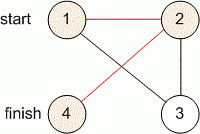Competitions

# The shortest path

The not oriented graph is given. Find the shortest path from vertex a to vertex b.

#### Input

The first line contains two integers n and m (1n5 * 104, 1m105) - the number of vertices and edges. The second line contains two integers a and b - the starting and ending point correspondingly. Next m lines describe the edges.

#### Output

If the path between a and b does not exist, print -1. Otherwise print in the first line the length l of the shortest path between these two vertices in number of edges, and in the second line print l + 1 numbers - the vertices of this path.Time limit 2 seconds
Memory limit 128 MiB
Input example #1
4 5
1 4
1 3
3 2
2 4
2 1
2 3

Output example #1
2
1 2 4

Input example #2
4 4
2 3
2 1
2 4
4 3
1 3

Output example #2
2
2 1 3